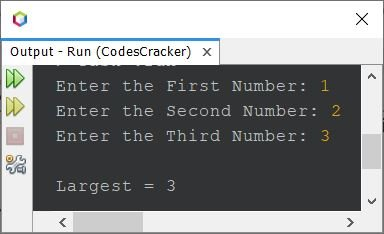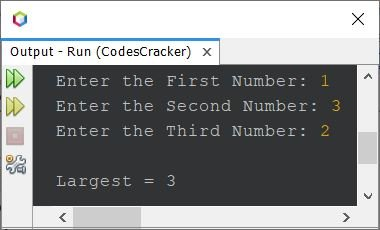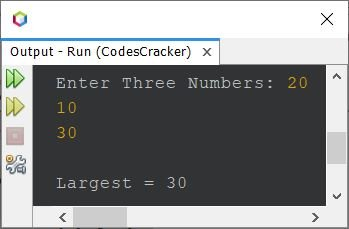# Java Program to Find Largest of Three Numbers

• Find largest of three numbers using if ... else
• Find largest of three numbers using nested if ... else
• Find largest of three numbers using conditional operator (?:)

## Find Largest of Three Numbers using if else

The question is, write a Java program to find the largest of three given numbers. The program given below is its answer:

```import java.util.Scanner;

public class CodesCracker
{
public static void main(String[] args)
{
int a, b, c, large;
Scanner scan = new Scanner(System.in);

System.out.print("Enter the First Number: ");
a = scan.nextInt();
System.out.print("Enter the Second Number: ");
b = scan.nextInt();
System.out.print("Enter the Third Number: ");
c = scan.nextInt();

if(a>b && a>c)
large = a;
else if(b>a && b>c)
large = b;
else
large = c;

System.out.println("\nLargest = " +large);
}
}```

The snapshot given below shows the sample run of above program, with user input 1, 2, and 3 as first, second, and third number to find and print the largest among these three numbers:Here is another sample run with user input 1, 3, and 2 as three numbers:## Find Largest of Three Numbers using Nested if else

This program is created by implementing the actual logic behind finding the largest number among three given numbers, using nested if...else

```import java.util.Scanner;

public class CodesCracker
{
public static void main(String[] args)
{
int a, b, c, large;
Scanner scan = new Scanner(System.in);

System.out.print("Enter Three Numbers: ");
a = scan.nextInt();
b = scan.nextInt();
c = scan.nextInt();

if(a>b)
{
if(b>c)
large = a;
else
{
if(c>a)
large = c;
else
large = a;
}
}
else
{
if(b>c)
large = b;
else
large = c;
}

System.out.println("\nLargest = " +large);
}
}```

Here is its sample run with user input 20, 10, and 30 as three numbers:## Find Largest of Three Numbers using Conditional Operator

This is the last program of this article, created using conditional operator or ternary operator, that is ?:. I've used the previous program, to wrap the if...else into conditional operator:

```import java.util.Scanner;

public class CodesCracker
{
public static void main(String[] args)
{
Scanner scan = new Scanner(System.in);

System.out.print("Enter Three Numbers: ");
int a = scan.nextInt();
int b = scan.nextInt();
int c = scan.nextInt();

int large = (a>b) ? ((b>c)?a:(c>a)?c:a) : (b>c)?b:c;

System.out.println("\nLargest = " +large);
}
}```

In the following statement, from above program:

`int large = (a>b) ? ((b>c)?a:(c>a)?c:a) : (b>c)?b:c;`

First the code (condition) a>b gets evaluated. If this code evaluates to be True, then the following code:

`((b>c)?a:(c>a)?c:a)`

will get evaluated, otherwise the following code:

`(b>c)?b:c`

will get evaluated. Let's suppose the condition a>b evaluates to be True. Therefore, the following code:

`((b>c)?a:(c>a)?c:a)`

will get evaluated in a way that the condition b>c gets evaluated. If this condition evaluates to be True, then

`a`

gets evaluated, otherwise:

`(c>a)?c:a)`

gets evaluated. This process continues until the whole expression lefts a single variable i.e., a, b, or c. Let's demonstrates the statement using the example given below.

#### How the Largest of Three Numbers gets Calculated using Conditional Operator

For example, if a=20, b=10, and c=30. Then the statement:

`int large = (a>b) ? ((b>c)?a:(c>a)?c:a) : (b>c)?b:c;`

gets evaluated as, first the code a>b or 20>10 evaluates to be True, therefore the code ((b>c)?a:(c>a)?c:a) gets evaluated.

That is, the code (b>c) or (10>30) evaluates to be False, therefore the code (c>a)?c:a gets evaluated.

That is, the code c>a or 30>20 evaluates to be True, therefore the code c gets evaluated. That is, no more code to execute, as the final variable is c, therefore the value of c, that is 30 will get initialized to large. That's it.

#### Same Program in Other Languages

Java Online Test

« Previous Program Next Program »